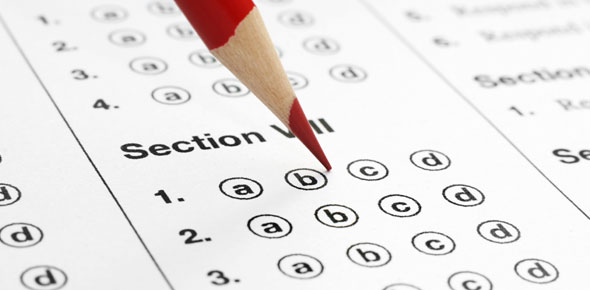# Wonderlic Sample Quiz: Can You Pass?

28 Questions | Attempts: 2338
ShareSettingsSample questions similar to those found on the Wonderlic exam are here for you. Do you think you can pass this Wonderlic sample quiz easily and get a good score? Well, we will see as you attempt this quiz. The Wonderlic Personnel Test measures your problem-solving skills as well as your ability to perform under pressure. Try to answer as many questions correctly as you can. We wish you good luck and hope you get a very high score. Let us just now go for it now!

• 1.
Round 907.457 to the nearest tens place
• A.

908.00

• B.

910.00

• C.

907.50

• D.

900.00

• E.

907.46

• 2.
The average of 12, 15, 23, 10
• A.

10

• B.

15

• C.

16

• D.

12

• E.

20

• 3.
On Monday and Thursday, the cost of lunch is \$5.43 total. On Tuesday and Wednesday the cost of lunch is \$3.54 on each day. On Friday, the cost of lunch is \$7.89. What was the average daily cost?
• A.

3.19

• B.

3.75

• C.

3.90

• D.

4.08

• E.

4.23

• 4.
1230.932567 rounded to the nearest hundredths place is
• A.

1200

• B.

1230.9326

• C.

1230.930

• D.

1230.00

• E.

1230.9330

• 5.
Subtract the following numbers rounded to the nearest tenths place. 134.679     45.548      67.8807
• A.

21.30

• B.

21.25

• C.

-58.97

• D.

-59.00

• E.

1.00

• 6.
What is the mathematical average of the number of weeks in a year, seasons in a year, and the number of days in January?
• A.

36

• B.

33

• C.

32

• D.

31

• E.

29

• 7.
Over the course of a week, Fred spent \$28.49 on lunch. What was the average cost per day?
• A.

4.07

• B.

3.57

• C.

6.51

• D.

2.93

• E.

5.41

• 8.
A roast was cooked at 325° F in the oven for 4 hours. The internal temperature rose from 32° F to 145° F. What was the average rise in temperature per hour?
• A.

20.2

• B.

28.25

• C.

32.03

• D.

37.0

• E.

37.29

• 9.
In the number 743.25 which digit represents the tenths space
• A.

2

• B.

3

• C.

4

• D.

5

• E.

7

• 10.
Add 0.98 + 45.102 + 32.3333 + 31 + 0.00009
• A.

368.573

• B.

210.536299

• C.

109.41539

• D.

99.9975

• E.

80.8769543

• 11.
WISH          ORDER
• A.

Similar

• B.

• C.

Not related

• 12.
Find 0.12 ÷ 1
• A.

12

• B.

1.2

• C.

.12

• D.

.012

• E.

.0012

• 13.
(9 ÷ 3) x (8 ÷ 4) =
• A.

1

• B.

6

• C.

72

• D.

576

• E.

752

• 14.
6 x 0 x 5 =
• A.

30

• B.

11

• C.

25

• D.

0

• E.

27

• 15.
7.95 ÷ 1.5 =
• A.

2.4

• B.

5.3

• C.

6.2

• D.

7.3

• E.

7.5

• 16.
-32 + 7 equals:
• A.

-25

• B.

25

• C.

-26

• D.

26

• E.

27

• 17.
-37 + -47 equals:
• A.

64

• B.

-84

• C.

65

• D.

-75

• E.

-66

• 18.
41% equals:
• A.

4.1

• B.

.41

• C.

.041

• D.

.0041

• E.

.00415

• 19.
Which of the following is not a whole number followed by its square?
• A.

1, 1

• B.

6, 36

• C.

8, 64

• D.

10, 100

• E.

11, 144

• 20.
Which number is largest?
• A.

32.000

• B.

23000

• C.

32.00

• D.

3.200

• E.

230.00

• 21.
Art realized that he had 2 more quarters than he had originally thought in his pocket. If all of the change in his pocket is quarters and it totals to \$8.75, how many quarters did he originally think were in his pocket?
• A.

27

• B.

29

• C.

31

• D.

33

• E.

35

• 22.
There are 12 more apples than oranges in a basket of 36 apples and oranges. How many apples are in the basket?
• A.

12

• B.

15

• C.

24

• D.

28

• E.

36

• 23.
Which of the following correctly identifies 4 consecutive odd integers where the sum of the middle two integers is equal to 24?
• A.

5, 7, 9, 11

• B.

7, 9, 11, 13

• C.

9, 11, 13, 15

• D.

11, 13, 15, 17

• E.

13, 15, 17, 19

• 24.
What is the next number in the sequence? 6, 12, 24, 48, ___
• A.

72

• B.

96

• C.

108

• D.

112

• E.

124

• 25.
A train travels 20 feet in 1/5 seconds At this exact speed, how many feet will it travel in three seconds?
• A.

60

• B.

150

• C.

200

• D.

300

• E.

500

## Related TopicsBack to top
×

Wait!
Here's an interesting quiz for you.